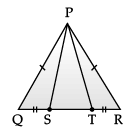Test: Triangles- Assertion-Reason & Case Base Type Questions

# Test: Triangles- Assertion-Reason & Case Base Type Questions - Class 9

Test Description

## 6 Questions MCQ Test Additional Practice for Class 9 - Test: Triangles- Assertion-Reason & Case Base Type Questions

Test: Triangles- Assertion-Reason & Case Base Type Questions for Class 9 2023 is part of Additional Practice for Class 9 preparation. The Test: Triangles- Assertion-Reason & Case Base Type Questions questions and answers have been prepared according to the Class 9 exam syllabus.The Test: Triangles- Assertion-Reason & Case Base Type Questions MCQs are made for Class 9 2023 Exam. Find important definitions, questions, notes, meanings, examples, exercises, MCQs and online tests for Test: Triangles- Assertion-Reason & Case Base Type Questions below.
Solutions of Test: Triangles- Assertion-Reason & Case Base Type Questions questions in English are available as part of our Additional Practice for Class 9 for Class 9 & Test: Triangles- Assertion-Reason & Case Base Type Questions solutions in Hindi for Additional Practice for Class 9 course. Download more important topics, notes, lectures and mock test series for Class 9 Exam by signing up for free. Attempt Test: Triangles- Assertion-Reason & Case Base Type Questions | 6 questions in 12 minutes | Mock test for Class 9 preparation | Free important questions MCQ to study Additional Practice for Class 9 for Class 9 Exam | Download free PDF with solutions
 1 Crore+ students have signed up on EduRev. Have you?
Test: Triangles- Assertion-Reason & Case Base Type Questions - Question 1

### Direction: A children‘s park is in the shape of isosceles triangle said PQR with PQ = PR, S and T are points on QR such that QT = RS.Q. Which rule is applied to prove that congruency of ΔPQS and ΔPRT.

Detailed Solution for Test: Triangles- Assertion-Reason & Case Base Type Questions - Question 1
In ΔPQS and ΔPRT

PQ = PR (Given)

QS = TR (Given)

∠PQR =∠PRQ (corresponding angles of an isosceles ∆)

By S AS congmency

∆PQS ≅ ∆PRT

Test: Triangles- Assertion-Reason & Case Base Type Questions - Question 2

### Direction: A children‘s park is in the shape of isosceles triangle said PQR with PQ = PR, S and T are points on QR such that QT = RS.Q. If ∠QPR = 80°. find ∠PQR?

Detailed Solution for Test: Triangles- Assertion-Reason & Case Base Type Questions - Question 2
let ∠Q = ∠R = x and ∠P = 80°

In ∆ PQR, ∠P + ∠Q + ∠R = 180° (Angle sum property of ∆)

80° + x + x = 180°

2x = 180° – 80

2x = 100°

x = 100°/2 = 50°

Test: Triangles- Assertion-Reason & Case Base Type Questions - Question 3

### Direction: A children‘s park is in the shape of isosceles triangle said PQR with PQ = PR, S and T are points on QR such that QT = RS.Q. In RHS rule ‘H‘ stands for :

Detailed Solution for Test: Triangles- Assertion-Reason & Case Base Type Questions - Question 3
‘H‘ stands for hypotenuse.
Test: Triangles- Assertion-Reason & Case Base Type Questions - Question 4

Direction: A children‘s park is in the shape of isosceles triangle said PQR with PQ = PR, S and T are points on QR such that QT = RS.Q. An isosceles triangle has

Detailed Solution for Test: Triangles- Assertion-Reason & Case Base Type Questions - Question 4
An isosceles ∆ has 2 sides equal.
Test: Triangles- Assertion-Reason & Case Base Type Questions - Question 5

Direction: A children‘s park is in the shape of isosceles triangle said PQR with PQ = PR, S and T are points on QR such that QT = RS.Q. If PQ = 6 cm and QR = 7 cm, then perimeter of ΔPQR is :

Detailed Solution for Test: Triangles- Assertion-Reason & Case Base Type Questions - Question 5
Perimeter = sum of all 3 sides

PQ = PR = 6 cm,

QR = 7 cm

So P = (6 + 6 + 7) cm

= 19 cm

Test: Triangles- Assertion-Reason & Case Base Type Questions - Question 6

Direction: Assertion
Statement 1: In triangle ABC, the centroid (G) divides the line joining orthocentre (O) and circumcentre (C) in ratio 2 : 1.
Reason
Statement 2: The centroid (G) divides the median AD in ratio 2 : 1. Select the correct

Detailed Solution for Test: Triangles- Assertion-Reason & Case Base Type Questions - Question 6

In the question, we have to check that the Statement 1: In triangle ABC, the centroid (G) divides the line joining orthocentre (O) and circumcentre (C) in ratio 2 : 1, is true or not and also we have to check that Statement 2: The centroid (G) divides the median AD in ratio 2 : 1, is correct or not. Finally, we have to check if statement 2 is the explanation of statement 1 or not.
So, here we know that In triangle ABC, the centroid (G) divides the line joining orthocentre (O) and circumcentre (C) in ratio 2 : 1. Also, we know that The centroid (G) divides the median AD in ratio 2 : 1. But the explanation given in statement 2 is not the correct reason for the statement 1 to be true.
So, from this we can say that option B, which says that Both the statements are TRUE but STATEMENT 2 is NOT the correct explanation of STATEMENT 1 is the correct answer.
Hence, option B is the correct answer.

## Additional Practice for Class 9

266 docs|65 tests
Information about Test: Triangles- Assertion-Reason & Case Base Type Questions Page
In this test you can find the Exam questions for Test: Triangles- Assertion-Reason & Case Base Type Questions solved & explained in the simplest way possible. Besides giving Questions and answers for Test: Triangles- Assertion-Reason & Case Base Type Questions, EduRev gives you an ample number of Online tests for practice

## Additional Practice for Class 9

266 docs|65 tests